Mathematical and Physical Journal
for High Schools
Issued by the MATFUND Foundation
 Already signed up? New to KöMaL?

# KöMaL Problems in Physics, April 2013

Show/hide problems of signs:## Problems with sign 'M'

Deadline expired on May 10, 2013.

M. 332. Fix a piece of thin flexible steel sheet (for example the blade of a knife or a saw) into a vise, such that at most 10 cm long part of it sticks out of the jaws of the vise. The end of the blade which sticks out can be round or pointed, it is not necessary to use a rectangular blade. Pluck the part of the blade which sticks out. Measure how the frequency f of the plucked blade (the pitch of the emitted sound) depends on the length of the midline of the oscillating part of the blade.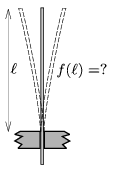(6 pont)

statistics## Problems with sign 'P'

Deadline expired on May 10, 2013.

P. 4529. The colour of coffee in a mug is black, but its foam is either white or cream-coloured. Why?

(3 pont)

solution (in Hungarian), statistics

P. 4530. A converging lens creates an upside-down image of height K=4 cm about an object of height T=2 cm. Determine the focal length of the lens with construction.

(3 pont)

solution (in Hungarian), statistics

P. 4531. How should a given amount of shots be distributed between the cart and the scale, in order that the tension in the string is to be the greatest? (The mass of the pulley is negligible.)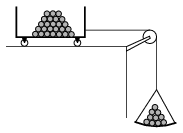(4 pont)

solution (in Hungarian), statistics

P. 4532. The bulbs connected as shown in the figure are alike, their power-rating is P when the voltage across them is U. What are the values of the voltage and the power across them, in case of open (ny) and closed (z) switches? Fill in the table.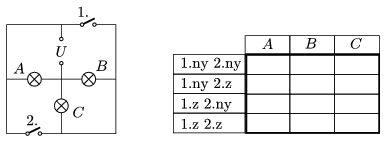(4 pont)

solution (in Hungarian), statistics

P. 4533. A student standing on the moving bus did not hold on to anything, and fell off when the bus took a bend. At what angle should he have to lean in order not to fall off, if the bus travelled at a speed of 30 km/h in the bend of radius 25 m?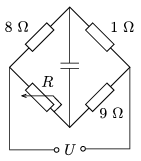(4 pont)

solution (in Hungarian), statistics

P. 4534. What is the value of the resistance of the variable resistor R shown in the figure, when

a) the condenser is uncharged;

b) the voltage across the condenser is exactly half of U?(4 pont)

solution (in Hungarian), statistics

P. 4535. The attached photograph (which can also be seen in the first cover of this journal) shows a phase of the descending Moon. Using the sizes of the figure determine how many days before the picture was taken was a full Moon.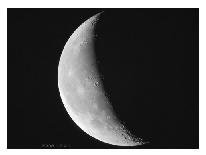(4 pont)

solution (in Hungarian), statistics

P. 4536. The theoretical limit of the efficiency of wind turbines is the so called Betz-limit is 59.3%. There are other losses, but the most modern wind turbines efficiency is approximately 84% of the Betz-limit.

a) How many megajoule kinetic energy is carried during 1 second by an air flow of speed of 27 km/h to that wind turbine which is at a height of 100 m, and which has 44 m-long blades?

b) What is the maximum output power of the turbine at this wind speed?

(4 pont)

solution (in Hungarian), statistics

P. 4537. I bought a cylinder shaped piece of cheese in a shop. At the cash-desk I put it onto the conveyor belt such that its horizontal symmetry axis was perpendicular to the rim of the conveyor belt. When the conveyor belt began to move the cheese also began to move. What was the speed of the centre of the cheese, if the speed of the conveyor belt was 60 cm/s?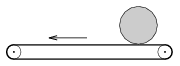(5 pont)

solution (in Hungarian), statistics

P. 4538. A frictionless slope, having a cross section of a quadrant of radius R=20 cm, is attached to a moveable cart as shown in the figure. The horizontal surface of the cart is rough, the coefficient of friction is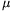=0.8. A point-like object falls freely from a height, measured from the plateau of the cart, of h=2R onto the top of the slope, and arrives tangentially. It slides along the path and just stops at the end of the cart. How long is the cart?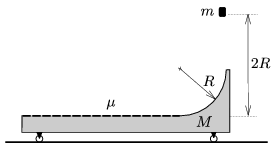(5 pont)

solution (in Hungarian), statistics

P. 4539. The base of a solid 4r-high right cylinder is a circle of radius r. One side of the surface of a tale made of hard but flexible material is slippery, whilst on the other side the frictional coefficient is. The two parts are separated by a straight line. The cylinder is placed to the slippery side of the table (with its circular base on the tabletop), and is given a push perpendicularly to the separation line. Will the cylinder fall when it reaches the separation line or not, and if yes how, if a=0.2; b=0.7; c=1.4? (The rotational inertia of a cylinder of mass m, radius of r and height of 4r about a horizontal axis through its centre of mass is.)

(6 pont)

solution (in Hungarian), statistics